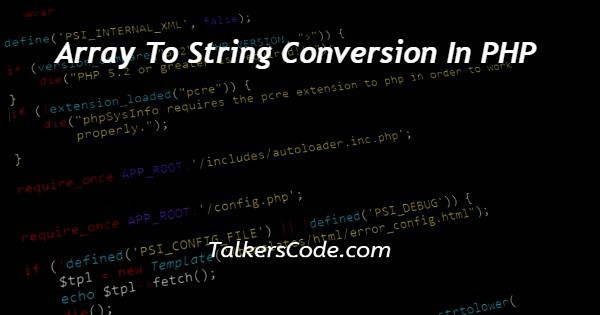# Array To String Conversion In PHP

Last Updated : Jan 1, 2023In this tutorial we will show you array to string conversion in PHP, this can be done in many ways. Mainly the need for conversion is because we cannot interact with arrays directly, so for better usage of data, we convert our arrays to strings and then do what we want.

Let us see the ways with help of we can convert arrays to strings using PHP.

## Step By Step Guide On Array To String Conversion In PHP :-

Now, the ways with help of which we can convert arrays to strings are:

• With the help of implode () function
• With the help of the join () function
• With the help of json_encode () function

In this tutorial, we are going to convert our first way that is with the help of implode () function.

The next two ways will be discussed in our next session. So, let us understand implode () function with the help of the example below.

But before that, we have to see the syntax of implode () function once.

```implode(separator,array)
<!DOCTYPE html>
<html>
<title> array to string conversion in PHP </title>
<body>
<h1>
TalkersCode
</h1>
<h2>
Array to string conversion in PHP
</h2>
<?php
\$string = array('Welcome','to','Talkers','Code');
// conversion of array to string using space
echo implode(" ",\$string)."<br>";
// conversion of array to string using # sign
echo implode("#",\$string)."<br>";
// conversion of array to string using - sign
echo implode("-",\$string)."<br>";
// conversion of array to string using X sign
echo implode("X",\$ string);
?>
</body>
</html>```
1. As, here we see that we that in above example we show you an example in which HTML and PHP codes are used.
2. Here, first of all, we create a basic structure of HTML, in which we use <!DOCTYPE html> which defines the type of document. And next one is our HTML tags. These tags are paired tags and all the data regarding HTML is written inside these tags.
3. After we use our head tag which is again paired tag and contains the title and meta information of the webpage. The data written inside the head is not shown on the webpage.
4. Now, next is the body which is the main tag of HTML. The data which we have written inside the body is shown on the webpage. Mostly all tags which are helpful to show data or information on the screen are written under the body tag.
5. Here, as we above see that we first created an array and store this inside a variable named string. Now, our job is to convert the array to string using PHP.
6. For this we use implode () function. As we know from the syntax of implode function that this requires a separator and array. In the example above, we give different separators and use the variables in which we store the array.
7. Now, all is done to see the output we open our codes in the browser using the server.

## Conclusion :-

At last, in conclusion, here we can say that with the help of this article we can understand how to convert an array to a string using PHP.

I hope this tutorial on array to string conversion in PHP helps you and the steps and method mentioned above are easy to follow and implement.